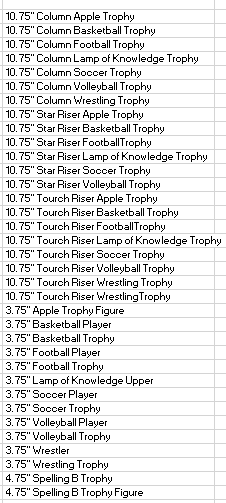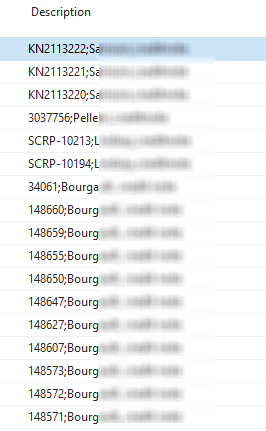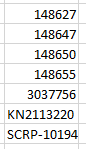Hi guys,

I want to filter part of a field which is separated by a wild character. In my scenario this is separated by ";".

Example:

BG_1234_P;Spare-parts

Filter based on the part of the field in bold.

In order to find the actual part of the field (in my case this is the "Description" field), I use the formula below:

=LEFT(I9;FIND(";";I9)-1)

The problem is incorporating this in my formula because, 1) I want to filter only part of the field which is easy as I use the formula above as my filtering value and 2) how do I reduce the data I want to be matched with in my field which is the hard part.

I have read the thread below that didn't work. Maybe because I'm trying to fit a nested excel formula in JET and messes up.

Thanks!

•Harry Lewis

Hello Jack -

If I understand correctly, this might be helpful.

Let's suppose that I am working in Dynamics NAV (required for this technique) and that I want all my Description values from the Item table where that field contains ".75" directly before an "inch" indicator (a double quote).

Here is that function:

=NL("Rows","Item","Description","=IFERROR(MID(NF(,""Description""),FIND("""""""",NF(,""Description""))-3,3),"""")",".75")

Let's look at the MID() function.

We're looking at the Description field.  We find the location of the double quote mark and look at the three characters before that.

If those three characters are ".75" we have a match.

I included the IFERROR to cover the potential error condition where the double quote is not present at all or appears before the 4th character in the description.

In my sample CRONUS database, the results are:•Jack

Many thanks Harry for your input.

I don't know the length of the string I want to filter with and that's why  I'm using both Find and Left formulas. Can I use both in my scenario?

•Jack

Any ideas how I can incorporate this with dual formula like the one in my comment above?

•Harry Lewis

Hello Jack -

I guess I do not fully understand what you are trying to do.

Can you show a mockup of what is in the tables and what you are trying to retrieve?

•Jack

Sure Harry. Let me explain this in more detail.

We are using the description field to record some specific transaction details. I then use jet reports to match customer with vendor transactions (many to many relationship, so I'm using sum of' in my formula).

These are the left part of the description field separated by semicolon. The length varies and that is why I'm using LEFT and FIND formulas.•Harry Lewis

And then you want to get data from another table where a field might look like this?•Jack

The other table will have the data in a similar format as the source table. So eventually I will be matching partial field with partial from another field.

I hope this helps.

•Jack

Any ideas how to incorporate this formula in JET?